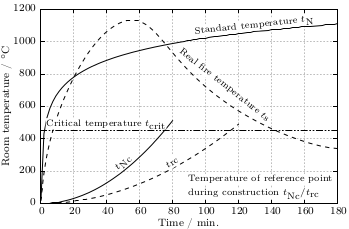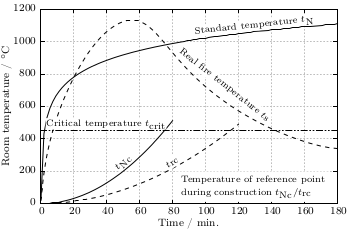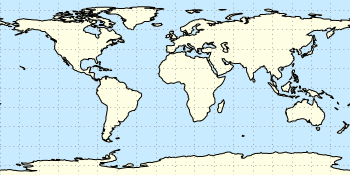# Gnuplotting

Create scientific plots using gnuplot

April 13th, 2015 | 3 Comments

Instead of using a legend it is often a good idea to label your data directly in the graph. If you use a grid it can happen that you want to use a white background with your labels. This would improve the readability of the labels as it reduces interaction with the grid. To add a background is not straightforward, especially if you have rotated labels. In the following, we will have a look how to solve the problem for LaTeX terminals. Thanks to V. Mózer for the idea and the data for the plot. Fig. 1 presents the desired result.Fig. 1 Fire severity as given by the fire temperature over time for a real vs. normalized fire. Click on the figure to see the original PDF version. (code to produce this figure, data)

To add a background to the labels we use the `colorbox` command, which we include in our terminal definition via the `header` option.

```set terminal cairolatex standalone pdf size 16cm,10.5cm dashed transparent \
```

In addition, we specify the size of the background area with the `\setlength{\fboxsep}{0.75pt}` command. This is quite handy as the default background size of `\colorbox` is a little to large for labels.

For the labels themselves, we only have to highlight them with the `\hl{}` command to get the desired background.

```set label 1 at  50, 250 '\hl{\small \$t_\textrm{Nc}\$}' center rotate by 45 front
```Fig. 2 Fire severity as given by the fire temperature over time for a real vs. normalized fire. Click on the figure to see the original PDF version.(code to produce this figure, data)

If you have a label with a line break, you have to decide if you want to apply the background to every part of the line break, as shown in Fig. 1

```set label 2 at  90, 100 '\small \shortstack[l]{\hl{Temperature of reference '.\
'point} \\ \hl{during construction \$t_\textrm{Nc} / '.\
't_\textrm{rc}\$}}' front
```

or if you want to highlight the whole label without seeing some grid between the lines

```set label 2 at  90, 100 '\hl{\small \shortstack[l]{Temperature of '.\
'reference point \\ during construction '.\
'\$t_\textrm{Nc} / t_\textrm{rc}\$}}' front
```

Fig. 2 shows the result for that one.

April 3rd, 2013 | 3 Comments

In one of the last posts, we came up with an updated data set representing the world. One way to plot this data set is with a 2D plot, as shown in Fig. 2. But if you compare the output with the one you see for example at Google Maps you will noticed a difference. That is due to the fact that Google uses the Mercator projection of the data. This projection preserves the angles around any point on the map, what is useful if you have a close look at some streets. The disadvantage of the Mercator projection is the inaccuracy of the sizes of the countries near to the poles. For example the size of Greenland is completely overemphasized as you can see in Fig. 1.Fig. 1 Mercator projection of the world (code to produce this figure, data)

In order to achieve the Mercator projection, we apply the following function.

```set angles degrees
mercator(latitude) = log( tan(180/4.0 + latitude/2.0) )
set yrange [-3.1:3.1]
plot 'world_110m.txt' u 1:(mercator(\$2)) w filledcu ls 2
```Fig. 2 Equirectangular projection of the world (code to produce this figure, data)

By just plotting the data as we have done for Fig. 2, we have the Equirectangular projection with constant spacing between the latitudes and meridians. The blue background color in the first two figures can be achieved directly with a terminal setting.

```set terminal pngcairo size background '#c8ebff'
```Fig. 3 Mapping of the Mercator projection (code to produce this figure)

In Fig. 3 the Mercator projection function is shown as an input-output-function of the latitude values. The placing of the latitude values on the y-axis can be easily done with a loop.

```set ytics 0
do for [angle=-80:80:20] {